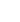## Errata forIntroduction to Electroacoustics and Audio Amplifier DesignbyW. Marshall Leach, Jr.

In the following, the star symbol (*) is used to indicate a multiplication, the forward slash (/) is used to indicate a division, the underline symbol ( _ ) is used to indicate a subscript, and the carat symbol (^) is used to indicate a superscript. Greek letters are spelled out.

### Second Edition, Revised Printing (copyright 1998, 1999, 2001) Errata

• Page 13, Equations (1.15) and (1.16). Change V0 to V02.
• Page 47. Last paragraph. Change "ZA = jωMA" to "ZA = jωMAω - 1/(jωCA)".
• Page 70. Problem 6. Part (a). In the answer, change "x0" to "x02".
• Page 138. Table 7.3. Column 1. Third line from bottom. Change "ESDPS" to "ESDPD".
• Page 145. Equation (8.18). This equation is valid only for the low frequencies where LE is a short circuit. Otherwise, "R¢E||(LEs)" must be added to the equation.
• Page 160. Problem 3. Part (b). Change "QES = 0.430" and "η0 = 0.545%" to "QES = 0.468" and "η0 = 0.500%".
• Page 162. Problem 9. Part (a) Change "5.67" to "5.26", change "0.0312" to "5.82", and change "0.177" to "3.41".
• Page 162. Problem 10. Part (a) answers: "1.53, 2.79, and 2.12". Part (b) answers: "0.607, 0.267, 0.674, and 0.482".
• Page 182. Paragraph above Eq. (9.43). Change "2.5 mH" to "3 mH". Change "1 ohm" to "0.9 ohm".
• Page 200. Eq. (10.51). Change "ω3" to "ω32".
• Page 202. Step 9 on upper half of page. Change "CAF = 8.97 x 10-6" to "CAF = 8.97 x 10-9".
• Page 202. Step 10. Change "476" to "376".
• Page 204. Problem 5. Change "15 cm" to "1.5 cm".
• Page 204. Problem 5. Part (d). Change "peak" to "peak-to-peak".
• Page 214. Eq. (11.32). Change "1 + bAf" to "1 + bA".
• Page 215. Eq. (11.37). Change "AfV1/ω1f" to "Afω1fV1".
• Page 234. Paragraph 2, Line 3. Change "Fig. 11.24(a)" to "Fig. 11.23(a)".
• Page 260. Paragraph 1. The class-D amplifier can be traced to the early '50s, not to 1928. The negative feedback amplifier can be traced to as early as 1928.
• Page 271. Problem 2, part (c). Change "Af = 1000 and f1f = 1000" to "A = 1000 and f1 = 1000".
• Page 271. Problem 6, part (b). Change "new gain of the input stage" to "new dc gain constant of the amplifier".

#### Second Edition (copyright 1998, 1999) Errata

• Page ix. Second line. Delete "has".
• Page 29. Equation (2.45). Change "1/r" to "1/r2" and "r" to "r2".
• Page 37. Equation (2.67). Delete the first r′ under the integral in the middle line.
• Page 79. Figure 4.10. Change the directions of the arrows for "u" and "i".
• Page 87. Figure 4.22(b). Change "E+e1" to "E+e2".
• Page 91. Problem 3. Change "1:n" to "n:1" on the figure.
• Page 91. Problem 6(a). Change x0 to x02.
• Page 95. Second line from bottom. Change "p_02" to 2p_02".
• Page 96. Line 2. Change "Chapter 2" to "Chapter 3".
• Page 145. Equation (6.62). Change "w^4" to "w_u2^4".
• Page 188. Equation (8.33). Change to f_n = (f_el/2){2+[2+2(2+1/epsilon^2)^1/2]^1/2}^1/2.
• Page 193. Line one. Change "which is be less than f_B" to "which is less than f_B".
• Page 197. Line 2. Change "problems in Chapter 2" to "problem 12 in Chapter 3".
• Page 199. Problem 3. Part (a). Change 38.0 to 37.9, 0.552 to 0.551, 1.78 to 1.80, and 0.484 to 0.485. Part (b). Change 0.468 to 0.430 and 0.483 to 0.545. Part (c). Change 0.469 to 0.484.
• Page 209. Paragraph 2. Delete the first sentence.
• Page 297. Equation (11.71). Change the left "=" sign to a multiplication sign.
• Page 223. Line 2 from bottom. Change "Fig. 9.19" to "Fig. 9.20".
• Page 230. Equation (10.4). Delete the "+" sign.
• Page 235. Table 10.1. Change the six occurances of "/SQRT" to "/(SQRT".
• Page 244. Eq. (10.48). Change "ω3" to "ω32".
• Page 246. Step 9. Change "C_AF = 8.97 x 10^-6" to "C_AF = 8.97 x 10^-9".
• Page 246. Step 10. Change "476" to "376".
• Page 274. Line 4. Change "emitter applied" to "emitter is applied".
• Page 348. Equations "1.4" through "1.11" should be labeled "G.4" through "G.11".
• Page 348. In Eq. (1.11), which should be labeled (G.11), change the 1/6 to 1/n, where n = 5 for Sec. 1.1.2 and n = 6 for Sec. G.2.2.
• Page 349. Eq. (G.12). Change "epsilon^2" to "epsilon".
• Page 350. Sec. G.2.2. To calculate k, use n = 6 in Eq. (1.11), which should be labeled (G.11).
• Page 351. Last line of paragraph 2. Change "f_S = 42.17" to "f_S = 67.4". Change "f_B = hf_S = 30.1" to "f_B = hf_S = 48.1".
• Page 360. Paragraph 2. Change "diaphragm and suspension" to "diaphragm suspension".
• Page 361. Paragraph 2. Change "f/f_0" to "frequency".

####First Edition (copyright 1998) ErrataErrors in the book will be posted to to the errata as they are found. If anyone finds an error that is not listed, I can be contacted at:This page is not a publication of the Georgia Institute of Technology and the Georgia Institute of Technology has not edited or examined the content. The author of this page is solely responsible for the content.